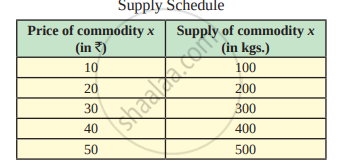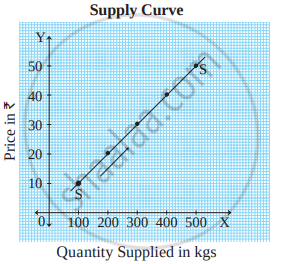# Assumptions of the Law of Supply

## Notes

#### Assumptions of the law :

The law of supply is based on the following assumptions :
1) Constant cost of production :
It is assumed that there is no change in the cost of production .A change in cost of production will affect the profits of the seller. Therefore less quantity will be supplied at the same price.
2) Constant technique of production :
It is also assumed that technique of production does not change. Improved technique of production may lead to an increase in production. This in turn may lead to an increase in the supply at the same price.
3) No change in weather conditions :
It is assumed that there is no change in the weather conditions. Natural calamities like floods, earthquakes etc. may decrease supply.
4) No change in Government policy :
It is also assumed that government policies like taxation policy, trade policy etc. remain unchanged.
5) No change in transport cost :
It is assumed that there is no change in the condition of transport facilities and transport cost. For example, better transport facility increases supply at the same price.
6) Prices of other goods remain constant :
Prices of other goods are assumed to remain constant. If they change, the law of supply may not hold true because producer may transfer resources to other products.
7) No future expectations :
The law also assumes that the sellers do not expect future changes in the price of the product.

Law of supply is explained with the help of the following schedule and diagram :It explains the direct relationship between price and quantity of commodity supplied. When price rises from ₹10 to ₹20, 30,40 and 50, the supply also rises from 100 to 200,300, 400 and 500 units respectively. It means, when price rises supply also rises and when price falls supply also falls. Thus, there is direct relationship between price and quantity supplied which is shown in following figure:In the figure above, X axis represents quantity supplied and Y axis represents the price of the commodity. Supply curve 'SS' slopes upwards from left to right which has a positive slope. It indicates a direct relationship between price and quantity supplied.

If you would like to contribute notes or other learning material, please submit them using the button below.